## What is the kinetic energy of a 108 kg object that is moving with a speed of 11.1 m/s ?

Question

What is the kinetic energy of a 108 kg object that is moving with a speed of 11.1 m/s ?

in progress 0
2 weeks 2021-09-02T20:25:31+00:00 1 Answers 0 views 0

6,653.34 J

Explanation:

Kinetic energy can be found using the following formula.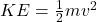where m is the mass in kilograms and v is the velocity in m/s.

The object is 108 kilograms and is moving at a speed of 11.1 m/s. Therefore, the mass is 108 kg and the velocity is 11.1 m/s.

m=108 kg

v=11.1 m/s

Substitute these values into the formula.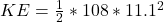Evaluate the exponent first. 11.1^2 is the same as 11.1*1.11, which is equal to 123.21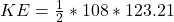Multiply 108 and 123.21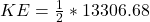Multiply 1/2 and 13306.68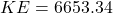The kinetic energy of the object is 6,653.34 Joules (kg m2/s^2)